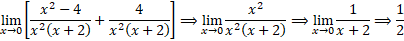It looks like you're using Internet Explorer 11 or older. This website works best with modern browsers such as the latest versions of Chrome, Firefox, Safari, and Edge. If you continue with this browser, you may see unexpected results.

# Math

Some of the content of this guide was modeled after a guide originally created by Openstax and has been adapted for the GPRC Learning Commons in September 2021. The graphs are generated using Desmos. This work is licensed under a Creative Commons BY 4.0 International License.## The Squeeze Theorem

The squeeze theorem states if: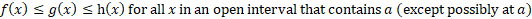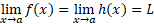Then: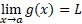Example: The Squeeze Theorem

The chart below shows three functions all converging on a point (0,1):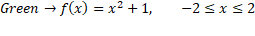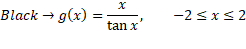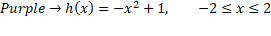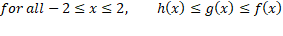To find the limit of g(x), use the limits of f(x) and h(x):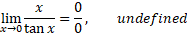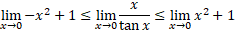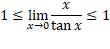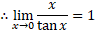## Alternate Examples of Limits

Example: Factoring and Canceling

Consider the function shown below.  The numerator and denominator both equate to zero resulting in an indeterminate limit.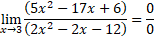To solve for this limit, the numerator and denominator can both be factored out and we find there is a common term of (x-3) that can be cancelled out, resulting in a form that can be solved for the limit.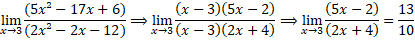Example: Multiplying by a Conjugate

Consider the function shown below.  The numerator and denominator both equate to zero resulting in an indeterminate limit.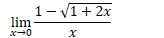To solve for this limit, we will multiply the top and the bottom of this equation by the conjugate of the numerator to remove the square root.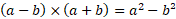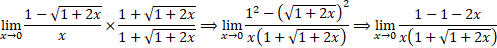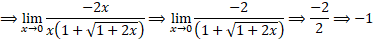Example: Combine and Simplify

Consider the function shown below.  Both parts of the function do not have a limit at zero.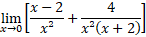To solve for this limit, we will combine the functions into one function and simplify the equation.